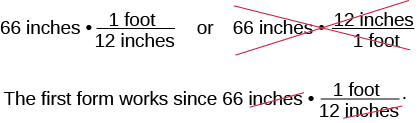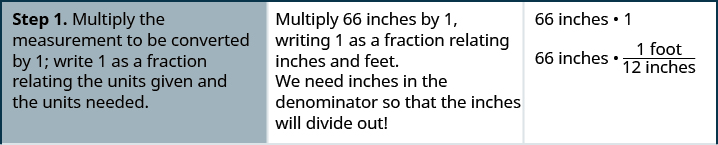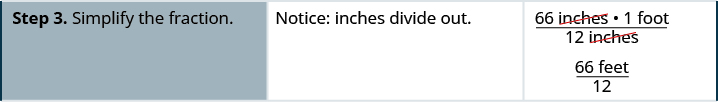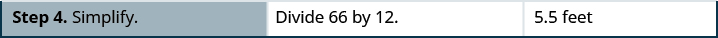# 1.10 Systems of measurement

 Page 1 / 13
By the end of this section, you will be able to:
• Make unit conversions in the US system
• Use mixed units of measurement in the US system
• Make unit conversions in the metric system
• Use mixed units of measurement in the metric system
• Convert between the US and the metric systems of measurement
• Convert between Fahrenheit and Celsius temperatures

A more thorough introduction to the topics covered in this section can be found in the Prealgebra chapter, The Properties of Real Numbers .

## Make unit conversions in the u.s. system

There are two systems of measurement commonly used around the world. Most countries use the metric system. The U.S. uses a different system of measurement, usually called the U.S. system . We will look at the U.S. system first.

The U.S. system of measurement uses units of inch, foot, yard, and mile to measure length and pound and ton to measure weight. For capacity, the units used are cup, pint, quart, and gallons. Both the U.S. system and the metric system measure time in seconds, minutes, and hours.

The equivalencies of measurements are shown in [link] . The table also shows, in parentheses, the common abbreviations for each measurement.

U.S. System of Measurement
$\begin{array}{ccc}\mathbf{\text{Length}}\hfill & & \begin{array}{ccc}1\phantom{\rule{0.2em}{0ex}}\text{foot}\phantom{\rule{0.2em}{0ex}}\text{(ft.)}\hfill & =\hfill & 12\phantom{\rule{0.2em}{0ex}}\text{inches}\phantom{\rule{0.2em}{0ex}}\text{(in.)}\hfill \\ 1\phantom{\rule{0.2em}{0ex}}\text{yard}\phantom{\rule{0.2em}{0ex}}\text{(yd.)}\hfill & =\hfill & 3\phantom{\rule{0.2em}{0ex}}\text{feet}\phantom{\rule{0.2em}{0ex}}\text{(ft.)}\hfill \\ 1\phantom{\rule{0.2em}{0ex}}\text{mile}\phantom{\rule{0.2em}{0ex}}\text{(mi.)}\hfill & =\hfill & 5,280\phantom{\rule{0.2em}{0ex}}\text{feet}\phantom{\rule{0.2em}{0ex}}\text{(ft.)}\hfill \end{array}\hfill \end{array}$ $\begin{array}{ccc}\mathbf{\text{Volume}}\hfill & & \begin{array}{ccc}3\phantom{\rule{0.2em}{0ex}}\text{teaspoons}\phantom{\rule{0.2em}{0ex}}\text{(t)}\hfill & =\hfill & 1\phantom{\rule{0.2em}{0ex}}\text{tablespoon}\phantom{\rule{0.2em}{0ex}}\text{(T)}\hfill \\ \text{16 tablespoons}\phantom{\rule{0.2em}{0ex}}\text{(T)}\hfill & =\hfill & \text{1 cup}\phantom{\rule{0.2em}{0ex}}\text{(C)}\hfill \\ \text{1 cup}\phantom{\rule{0.2em}{0ex}}\text{(C)}\hfill & =\hfill & \text{8 fluid ounces}\phantom{\rule{0.2em}{0ex}}\text{(fl. oz.)}\hfill \\ \text{1 pint}\phantom{\rule{0.2em}{0ex}}\text{(pt.)}\hfill & =\hfill & \text{2 cups}\phantom{\rule{0.2em}{0ex}}\text{(C)}\hfill \\ \text{1 quart}\phantom{\rule{0.2em}{0ex}}\text{(qt.)}\hfill & =\hfill & \text{2 pints}\phantom{\rule{0.2em}{0ex}}\text{(pt.)}\hfill \\ \text{1 gallon}\phantom{\rule{0.2em}{0ex}}\text{(gal)}\hfill & =\hfill & \text{4 quarts}\phantom{\rule{0.2em}{0ex}}\text{(qt.)}\hfill \end{array}\hfill \end{array}$
$\begin{array}{ccc}\mathbf{\text{Weight}}\hfill & & \begin{array}{ccc}\text{1 pound}\phantom{\rule{0.2em}{0ex}}\text{(lb.)}\hfill & =\hfill & \text{16 ounces}\phantom{\rule{0.2em}{0ex}}\text{(oz.)}\hfill \\ \text{1 ton}\hfill & =\hfill & \text{2000 pounds}\phantom{\rule{0.2em}{0ex}}\text{(lb.)}\hfill \end{array}\hfill \end{array}$ $\begin{array}{ccc}\mathbf{\text{Time}}\hfill & & \phantom{\rule{1em}{0ex}}\begin{array}{ccc}\text{1 minute}\phantom{\rule{0.2em}{0ex}}\text{(min)}\hfill & =\hfill & \text{60 seconds}\phantom{\rule{0.2em}{0ex}}\text{(sec)}\hfill \\ \text{1 hour}\phantom{\rule{0.2em}{0ex}}\text{(hr)}\hfill & =\hfill & \text{60 minutes}\phantom{\rule{0.2em}{0ex}}\text{(min)}\hfill \\ \text{1 day}\hfill & =\hfill & \text{24 hours}\phantom{\rule{0.2em}{0ex}}\text{(hr)}\hfill \\ \text{1 week}\phantom{\rule{0.2em}{0ex}}\text{(wk)}\hfill & =\hfill & \text{7 days}\hfill \\ \text{1 year}\phantom{\rule{0.2em}{0ex}}\text{(yr)}\hfill & =\hfill & \text{365 days}\hfill \end{array}\hfill \end{array}$

In many real-life applications, we need to convert between units of measurement, such as feet and yards, minutes and seconds, quarts and gallons, etc. We will use the identity property of multiplication to do these conversions. We’ll restate the identity property of multiplication here for easy reference.

## Identity property of multiplication

$\begin{array}{cccccc}\text{For any real number}\phantom{\rule{0.2em}{0ex}}a:\hfill & & & & & a·1=a\phantom{\rule{3em}{0ex}}1·a=a\hfill \\ 1\phantom{\rule{0.2em}{0ex}}\text{is the}\phantom{\rule{0.2em}{0ex}}\mathbf{\text{multiplicative identity}}\hfill & & & & & \end{array}$

To use the identity property of multiplication, we write 1 in a form that will help us convert the units. For example, suppose we want to change inches to feet. We know that 1 foot is equal to 12 inches, so we will write 1 as the fraction $\frac{1\phantom{\rule{0.2em}{0ex}}\text{foot}}{12\phantom{\rule{0.2em}{0ex}}\text{inches}}.$ When we multiply by this fraction we do not change the value, but just change the units.

But $\frac{12\phantom{\rule{0.2em}{0ex}}\text{inches}}{1\phantom{\rule{0.2em}{0ex}}\text{foot}}$ also equals 1. How do we decide whether to multiply by $\frac{1\phantom{\rule{0.2em}{0ex}}\text{foot}}{12\phantom{\rule{0.2em}{0ex}}\text{inches}}$ or $\frac{12\phantom{\rule{0.2em}{0ex}}\text{inches}}{1\phantom{\rule{0.2em}{0ex}}\text{foot}}?$ We choose the fraction that will make the units we want to convert from divide out. Treat the unit words like factors    and “divide out” common units like we do common factors. If we want to convert $66$ inches to feet, which multiplication will eliminate the inches?The inches divide out and leave only feet. The second form does not have any units that will divide out and so will not help us.

## How to make unit conversions

MaryAnne is 66 inches tall. Convert her height into feet.

## SolutionLexie is 30 inches tall. Convert her height to feet.

2.5 feet

Rene bought a hose that is 18 yards long. Convert the length to feet.

54 feet

integer greater than 2 and less than 12
2 < x < 12
Felix
I'm guessing you are doing inequalities...
Felix
Actually, translating words into algebraic expressions / equations...
Felix
hi
Darianna
hello
Mister
He charges $125 per job. His monthly expenses are$1,600. How many jobs must he work in order to make a profit of at least $2,400? Alicia Reply at least 20 Ayla what are the steps? Alicia 6.4 jobs Grahame 32 Grahame 1600+2400= total amount with expenses. 4000/125= number of jobs needed to make that min profit of 2400. answer is 32 Orlando He must work 32 jobs to make a profit POP what is algebra Azhar Reply repeated addition and subtraction of the order of operations. i love algebra I'm obsessed. Shemiah hi Krekar One-fourth of the candies in a bag of M&M’s are red. If there are 23 red candies, how many candies are in the bag? Leanna Reply they are 92 candies in the bag POP rectangular field solutions Navin Reply What is this? Donna t muqtaar the proudact of 3x^3-5×^2+3 and 2x^2+5x-4 in z7[x]/ is anas Reply ? Choli a rock is thrown directly upward with an initial velocity of 96feet per second from a cliff 190 feet above a beach. The hight of tha rock above the beach after t second is given by the equation h=_16t^2+96t+190 Usman Stella bought a dinette set on sale for$725. The original price was $1,299. To the nearest tenth of a percent, what was the rate of discount? Manhwa Reply 44.19% Scott 40.22% Terence 44.2% Orlando I don't know Donna if you want the discounted price subtract$725 from $1299. then divide the answer by$1299. you get 0.4419... but as percent you get 44.19... but to the nearest tenth... round .19 to .2 and you get 44.2%
Orlando
you could also just divide $725/$1299 and then subtract it from 1. then you get the same answer.
Orlando
p mulripied-5 and add 30 to it
Tausif
Tausif
how
muqtaar
Can you explain further
p mulripied-5 and add to 30
Tausif
-5p+30?
Corey
p=-5+30
Jacob
How do you find divisible numbers without a calculator?
TAKE OFF THE LAST DIGIT AND MULTIPLY IT 9. SUBTRACT IT THE DIGITS YOU HAVE LEFT. IF THE ANSWER DIVIDES BY 13(OR IS ZERO), THEN YOUR ORIGINAL NUMBER WILL ALSO DIVIDE BY 13!IS DIVISIBLE BY 13
BAINAMA
When she graduates college, Linda will owe $43,000 in student loans. The interest rate on the federal loans is 4.5% and the rate on the private bank loans is 2%. The total interest she owes for one year was$1,585. What is the amount of each loan?
Sean took the bus from Seattle to Boise, a distance of 506 miles. If the trip took 7 2/3 hours, what was the speed of the bus?
66miles/hour
snigdha
How did you work it out?
Esther
s=mi/hr 2/3~0.67 s=506mi/7.67hr = ~66 mi/hr
Orlando
No; 65m/hr
albert
hello, I have algebra phobia. Subtracting negative numbers always seem to get me confused.
what do you need help in?
Felix
Heather
look at the numbers if they have different signs, it's like subtracting....but you keep the sign of the largest number...
Felix
for example.... -19 + 7.... different signs...subtract.... 12 keep the sign of the "largest" number 19 is bigger than 7.... 19 has the negative sign... Therefore, -12 is your answer...
Felix
—12
Thanks Felix.l also get confused with signs.
Esther
Thank you for this
Shatey
ty
Graham
think about it like you lost $19 (-19), then found$7(+7). Totally you lost just $12 (-12) Annushka I used to struggle a lot with negative numbers and math in general what I typically do is look at it in terms of money I have -$5 in my account I then take out 5 more dollars how much do I have in my account well-\$10 ... I also for a long time would draw it out on a number line to visualize it
Meg
practicing with smaller numbers to understand then working with larger numbers helps too and the song/rhyme same sign add and keep opposite signs subtract keep the sign of the bigger # then you'll be exact
Meg
hi
albert
Bruce drives his car for his job. The equation R=0.575m+42 models the relation between the amount in dollars, R, that he is reimbursed and the number of miles, m, he drives in one day. Find the amount Bruce is reimbursed on a day when he drives 220 miles
168.50=R
Heather
john is 5years older than wanjiru.the sum of their years is27years.what is the age of each
46
mustee
j 17 w 11
Joseph
john is 16. wanjiru is 11.
Felix
27-5=22 22÷2=11 11+5=16
JoyceBy OpenStaxBy Saylor FoundationBy Janet ForresterBy OpenStaxBy John GabrieliBy Richley CrapoBy OpenStaxBy Anh DaoBy Cath YuBy Robert Morris